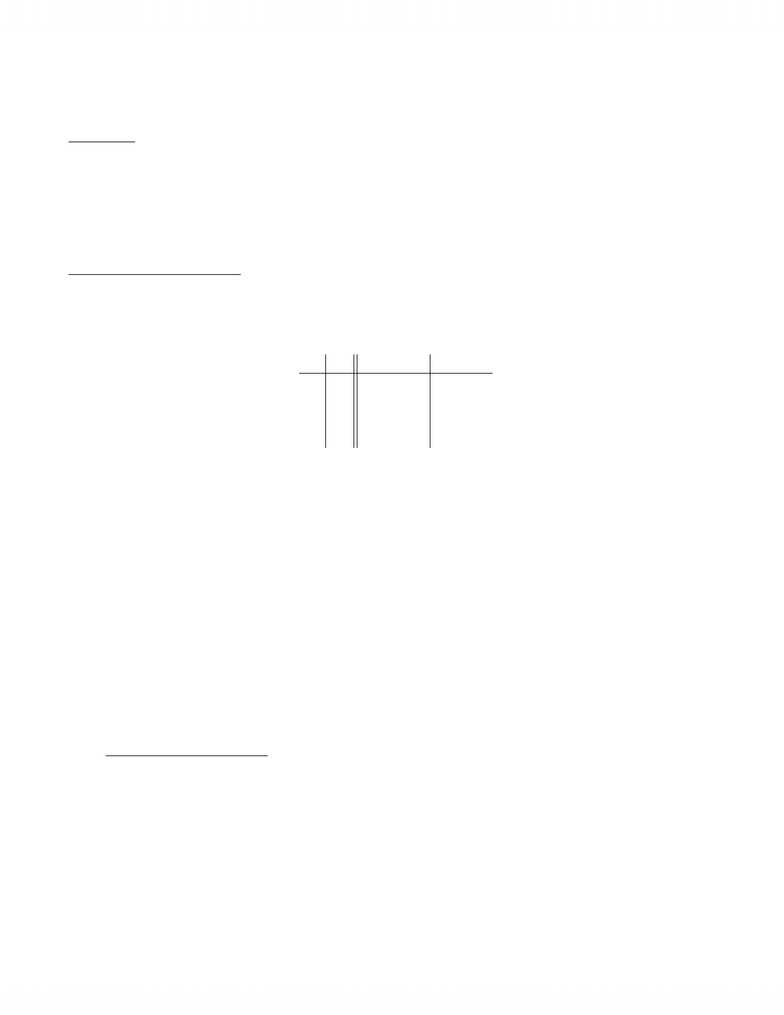# Lecture 3 notes Lecture 3 Winter 2009 notes including compound and converse statements

47 views3 pages
School
University of Waterloo
Department
Mathematics
Course
MATH135
ProfessorMATH 135 Winter 2009
Lecture III Notes
Notation
Some notation to remember:
Z={. . . 3,2,1,0,1,2,3, . . .}= set of integers
P={1,2,3,4, . . .}= set of positive integers
N.B. This notation is not standard, but is used by our textbook.
R= the set of all real numbers
Compound Statements
If Aand Bare mathematical statements, we often see compound statements such as “Aand B” and
Aor B”.
We deﬁne what the words “and” and “or” mean mathematically by using an organizational chart
called a truth table:
A B A and B A or B
T T T T
T F F T
F T F T
F F F F
Atruth table lists all possible combinations of TRUE (T) and FALSE (F) for the original statements
and tells us whether the compound statement is TRUE or FALSE in each case. (Truth tables can
be used to look at more complex compound statements, but we won’t do this in MATH 135.)
From the table, for “Aand B” to be TRUE, both Aand Bmust be TRUE.
Otherwise (when one is FALSE or both are FALSE), “Aand B” is FALSE.
For “Aor B” to be TRUE, either or both of Aand Bmust be TRUE.
Otherwise (when both are FALSE), “Aor B” is FALSE.
This makes sense when we consider the normal English language usage of these words.
Example
A=“2 is a prime number”, B=“5 is a perfect square”
Is “Aand B” TRUE or FALSE?
Is “Aor B” TRUE or FALSE?
Aside Regarding Sets
Recall that if Aand Bare sets, then ABis the set of elements that are in either Aor
B, and ABis the set of elements that are in both Aand B.
So ABis similar to “Aor B”, and ABis similar to “Aand B”.
Example
If A={1,2,4,5,6,9,10}and B={2,3,6,7,8}, then
AB={1,2,3,4,5,6,7,8,9,10}
AB={2,6}
Also, the statement NOT Ais called the negation of A. (With the example above, NOT Ais “2 is
not a prime number.) If statement Pis TRUE, then NOT Pis FALSE, and vice versa.
Unlock document

This preview shows page 1 of the document.
Unlock all 3 pages and 3 million more documents.